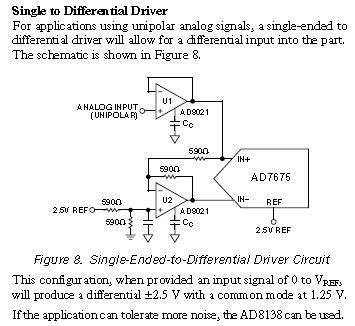# Single-ended to Differential Driver for Differential ADC

Status
Not open for further replies.

#### ericmar

##### Full Member level 5Dear All,However, I do not quite understand what it means by "This configuration, when provided an input signal of 0 to Vref, will produce a differential ±2.5V with a common mode at 1.25V."
1. Why is the differential ±2.5V and the common mode 1.25V?
2. What if the reference voltage is not 2.5V but 1.5V?
3. What happen if the +Input signal of U2 is not the same as the Reference voltage supplied to the AD7675? How does it affect everything?
4. Notice that one end of the 2nd 590Ω resistor at the +Input signal of U2 is connected to different ground as others. Why is it so?
5. How to calculate the resistance value?

By the way, my design is to convert a single-ended output signal, which is from a DAC, to a differential input ADC.

Regards,
Eric

#### bbbb

##### Member level 2Hi,

when in=2.5V then IN+=2.5V and IN-=0
So as in changes from 0 to 2.5V, (IN+ - IN- ) moves from -2.5V to 2.5V this is referred to as ±2.5v swing.
common mode level is the middle point on both of the inputs (each swing from 0 to 2.5) so it must be 1.25V.

2. if both refereces (one for bias of U2 and one for ADC) is X then the swing will be ±X and CM level is X/2

3. the + input of U2 is at REF/2. as an example, suppose this is set at zero. then the CM mode level will be zero. when in changes from 0 to 2.5 , IN+ will change from 0 to 2.5V and IN- from -2.5V to 0V. then (IN+ - IN-) > 2.5V in some cases. Assuming the ref of the ADC to still be at 2.5V, a differential input higher than REF will be clipped (e.g. for IN+ - IN-)>2.5V the output code of the ADC will remain at 11..1.

4. resistor values is not very important (as long as they as equal) the minimum limit is set by the Max output current of the opamp and also the linearity of hte opamp as a function of the load, the max limit is set by noise generated by the resistors and the phase delay caused by the inverting amplfier (U2).

You can also use a transformer for single ended to Diff. converrion and vise versa.
See the attached as an example of transformer. The circuit is similar to what given in Fig.3 of :
http://www.edn.com/article/CA302234.html

### ericmar

points: 2

#### ericmar

##### Full Member level 5Hi,

Thanks a lot for answering my question.
However, I do have one more question. Suppose I could not control the reference voltage of the ADC, and I have 2 single-ended signals (1 DAC output low voltage signal, typically 0~10mV, another one from the output of an op-amp, typically 0~5V) and I want these 2 signals to be added together and output as differential signals, can I simply use the above configuration?

Can I just connect the output of the DAC to the Analog Input (In+) of U1 and the output from the op-amp to the In+ of U2?

Thanks,
Eric

Status
Not open for further replies.